# K-means Clustering and Voronoi Sets

· Big Data Zone · Interview
Save
6.61K Views

in the context of -means , we want to partition the space of our observations into classes. each observation belongs to the cluster with the nearest mean. here “nearest” is in the sense of some norm, usually the (euclidean) norm.

consider the case where we have 2 classes. the means being respectively the 2 black dots. if we partition based on the nearest mean, with the (euclidean) norm we get the graph on the left, and with the (manhattan) norm, the one on the right,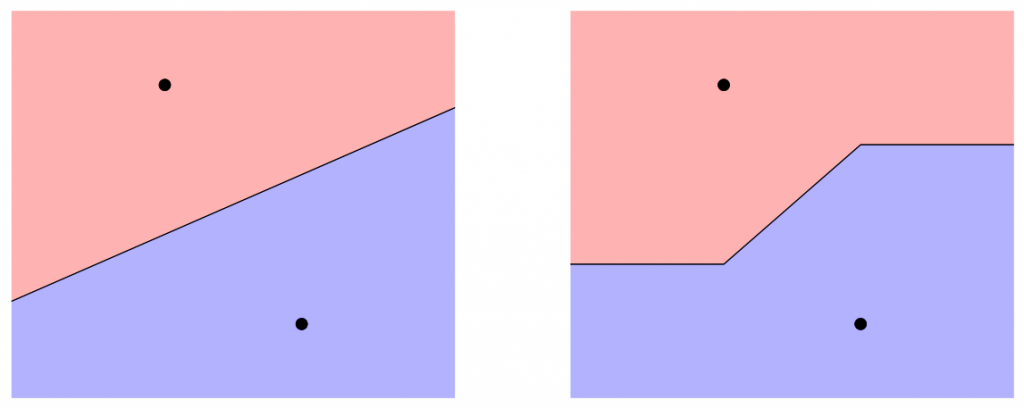points in the red region are closer to the mean in the upper part, while points in the blue region are closer to the mean in the lower part. here, we will always use the standard (euclidean) norm. note that the graph above is related to voronoi diagrams (or voronoy, from вороний in ukrainian, or вороно́й in russian) with 2 points, the 2 means.

in order to illustrate the -means clustering algorithm (here lloyd’s algorithm ) consider the following dataset

set.seed(1)
pts <- cbind(x=rnorm(500,rep(seq(1,9,by=2)/10,100),.022),y=rnorm(500,.5,.15))
plot(pts)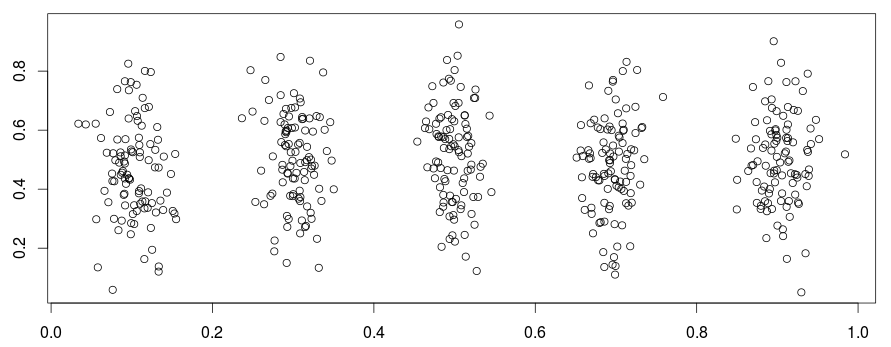here, we have 5 groups.  so let us run a 5-means algorithm here.

• we draw randomly 5 points in the space (intial values for the means),
• in the assignment step , we assign each point to the nearest mean

• in the update step , we compute the new centroids of the clusters

to visualize it, see:the code the get the clusters is:

kmeans(pts, centers=5, nstart = 1, algorithm = "lloyd")

observe that the assignment step is based on computations of voronoi sets. this can be done in r using:

library(tripack)
v <- voronoi.mosaic(means[,1],means[,2])
p <- voronoi.polygons(v)
points(v,pch=19)
plot(v,add=true)

this is what we can visualize below:the code to visualize the means, and the clusters (or regions), use:

km1 <- kmeans(pts, centers=5, nstart = 1, algorithm = "lloyd")
library(tripack)
library(rcolorbrewer)
cl5 <- brewer.pal(5, "pastel1")
v <- voronoi.mosaic(km1$centers[,1],km1$centers[,2])
p <- voronoi.polygons(v)
plot(pts,pch=19,xlim=0:1,ylim=0:1,xlab="",ylab="",col=cl5[km1$cluster]) points(km1$centers[,1],km1\$centers[,2],pch=3,cex=1.5,lwd=2)
plot(v,add=true)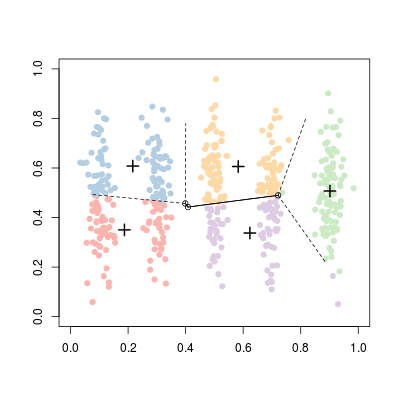here, starting points are draw randomly. if we run it again, we might get: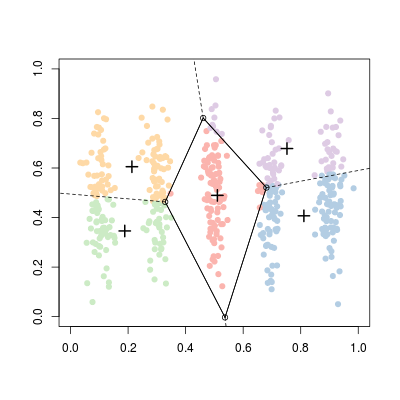or: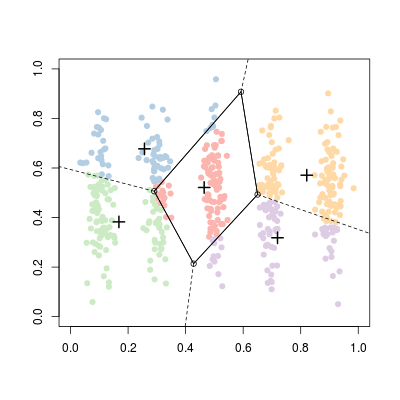on that dataset, it is difficult to get cluster that are the five groups we can actually see. if we use:

set.seed(1)
a <- c(rep(.2,100),rep(.2,100),rep(.5,100),rep(.8,100),rep(.8,100))
b <- c(rep(.2,100),rep(.8,100),rep(.5,100),rep(.2,100),rep(.8,100))
pts <- cbind(x=rnorm(500,a,.075),y=rnorm(500,b,.075))

we usually get something better.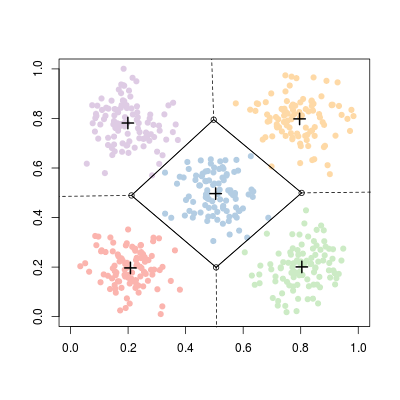colors are obtained from clusters of the -means function, but additional lines are obtained using as outputs of voronoi diagrams functions.

Topics:

Published at DZone with permission of Arthur Charpentier, DZone MVB.

Opinions expressed by DZone contributors are their own.